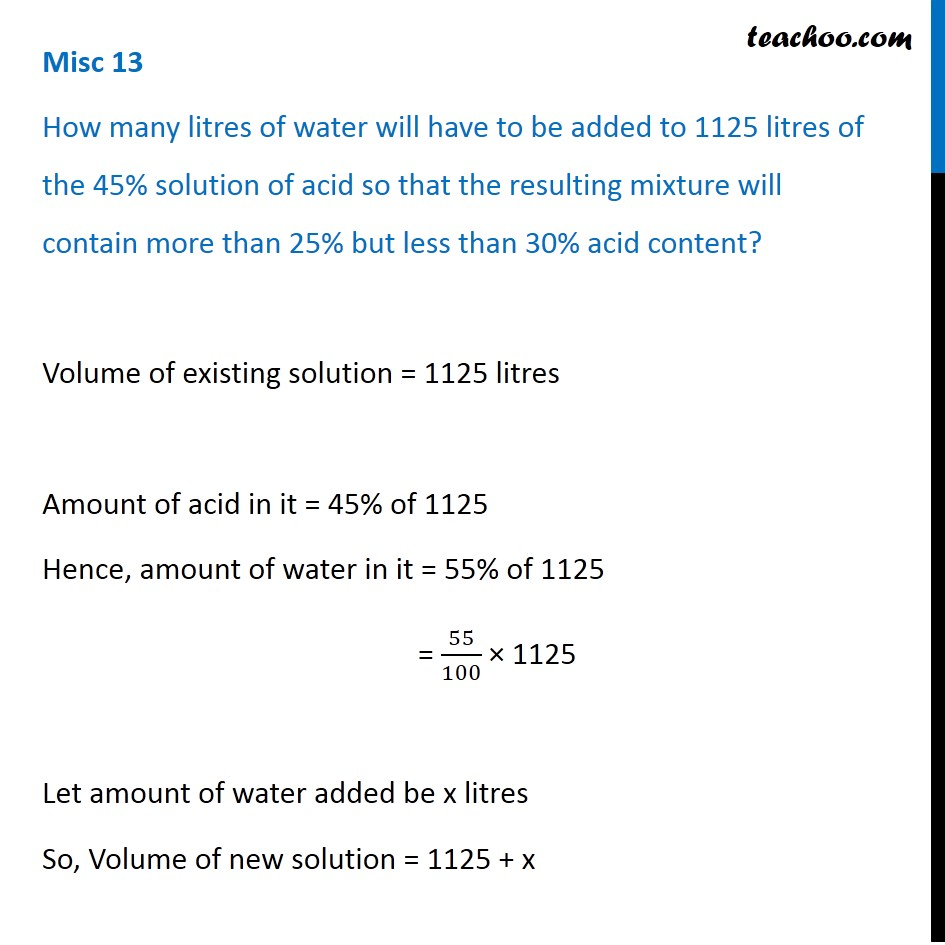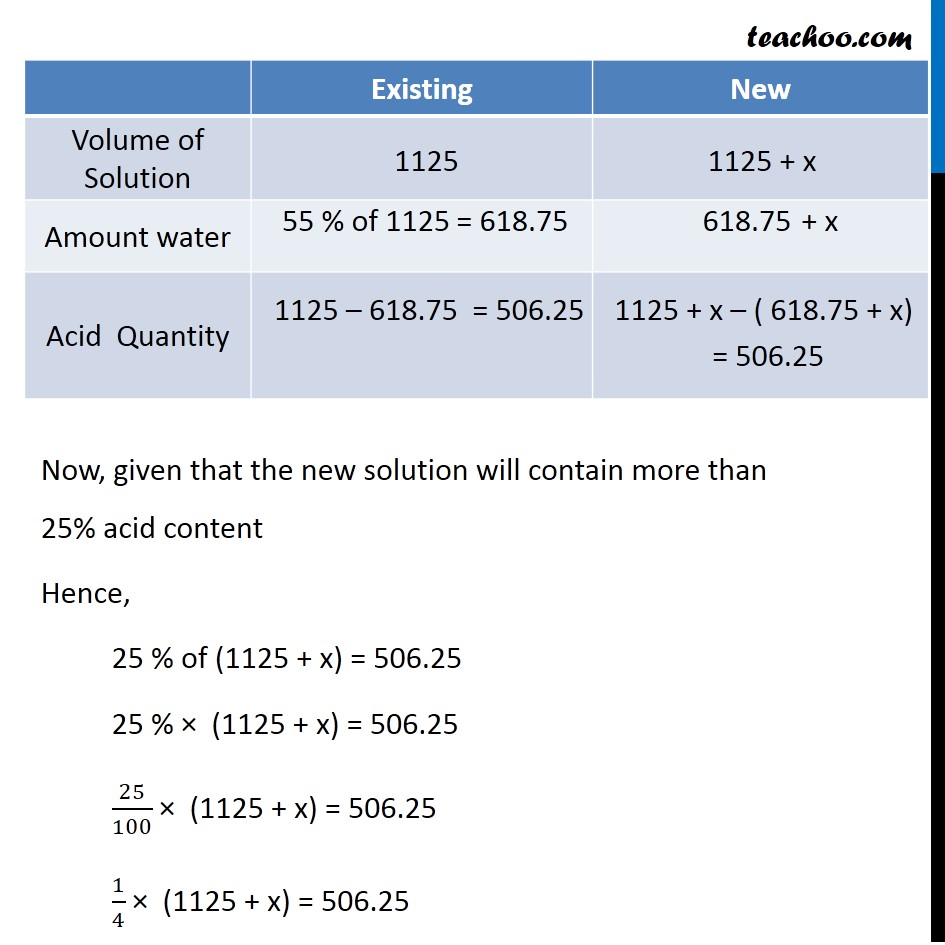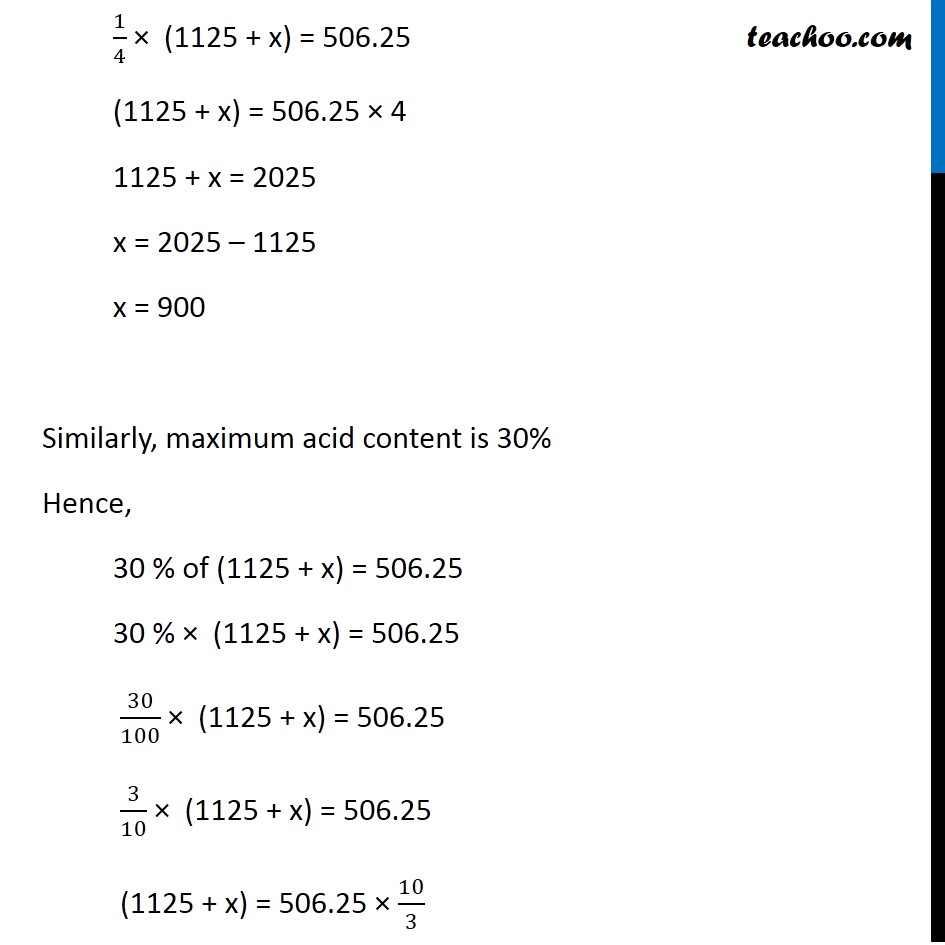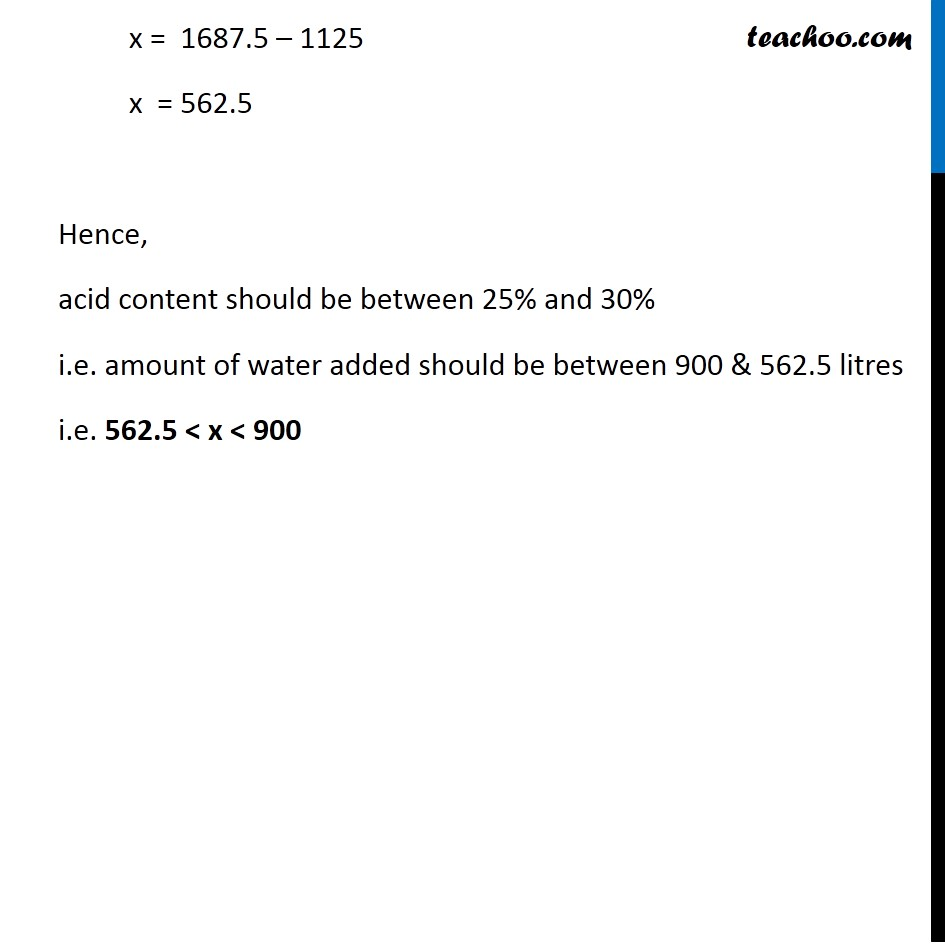Miscellaneous

Chapter 5 Class 11 Linear Inequalities
Serial order wiseLearn in your speed, with individual attention - Teachoo Maths 1-on-1 Class

### Transcript

Misc 13 How many litres of water will have to be added to 1125 litres of the 45% solution of acid so that the resulting mixture will contain more than 25% but less than 30% acid content? Volume of existing solution = 1125 litres Amount of acid in it = 45% of 1125 Hence, amount of water in it = 55% of 1125 = 55/100 × 1125 Let amount of water added be x litres So, Volume of new solution = 1125 + x Now, given that the new solution will contain more than 25% acid content Hence, 25 % of (1125 + x) = 506.25 25 % × (1125 + x) = 506.25 25/100 × (1125 + x) = 506.25 1/4 × (1125 + x) = 506.25 x = 1687.5 – 1125 x = 562.5 Hence, acid content should be between 25% and 30% i.e. amount of water added should be between 900 & 562.5 litres i.e. 562.5 < x < 900